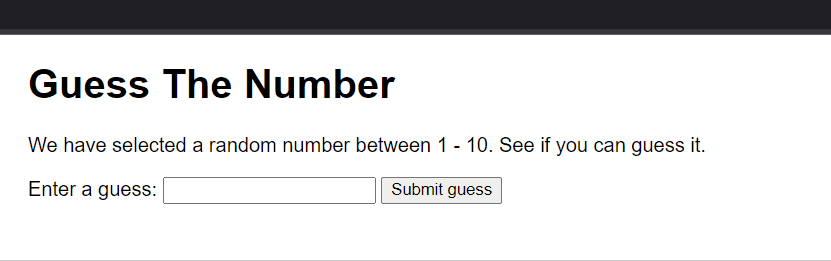Open In App

# Number Guessing Game using JavaScript

In this article, we will create a Number guessing game using JavaScript. The game is to guess a random number generated by a computer in the range 1 – 10 in a minimum number of Guesses.

Prerequisites:

Used Methods:

• document.getElementById(“given_id”) Method: The document.getElementById() method is used to fetch an element from the HTML page having the id as provided (specified) by the user. The “.value” is used to access the value of the HTML element accessed.
• JavaScript Math.random() Method: The random() method is used to generate a random number between 0 (inclusive) and 1 (exclusive). This generated number is then multiplied by 10 and added 1 to generate numbers from 1 to 10.
• JavaScript Math.floor() Method: The floor() method is used to return the number to the nearest integer (downwards). The value will not be rounded if the passed argument is an integer.

Implementation of the Game:

## HTML

 ```<``html``>``<``head``>``    ``<``meta` `charset``=``"utf-8"``>``    ``<``title``>Number Guessing Game` `    ``<``style``>``        ``body {``            ``font-family: sans-serif;``            ``width: 50%;``            ``max-width: 800px;``            ``min-width: 480px;``            ``margin: 0 auto;``        ``}``    ````` `<``body``>``    ``<``h1``>Guess The Number``    ``<``p``>``        ``We have selected a random number``        ``between 1 - 10. See if you can``        ``guess it.``    ``` `    ``<``div` `class``=``"form"``>``        ``<``label` `for``=``"guessField"``>``            ``Enter a guess:``        ````        ` `        ``<``input` `type``=``"text"` `id``=``"guessField"``            ``class``=``"guessField"``>``        ` `        ``<``input` `type``=``"submit"` `value``=``"Submit guess"``            ``class``=``"guessSubmit"` `id``=``"submitguess"``>``    ``` `    ``<``script` `type``=``"text/javascript"``>` `        ``// Generate a Random Number``        ``let y = Math.floor(Math.random() * 10 + 1);` `        ``// Counting the number of guesses``        ``// made for correct Guess``        ``let guess = 1;` `        ``document.getElementById("submitguess").onclick = function () {` `            ``// Number guessed by user   ``            ``let x = document.getElementById("guessField").value;` `            ``if (x == y) {``                ``alert("CONGRATULATIONS!!! YOU GUESSED IT RIGHT IN "``                    ``+ guess + " GUESS ");``            ``}` `            ``/* If guessed number is greater than actual number*/``            ``else if (x > y) {``                ``guess++;``                ``alert("OOPS SORRY!! TRY A SMALLER NUMBER");``            ``}``            ``else {``                ``guess++;``                ``alert("OOPS SORRY!! TRY A GREATER NUMBER")``            ``}``        ``}``    ```````

Output: